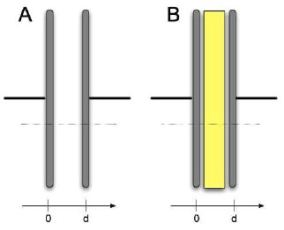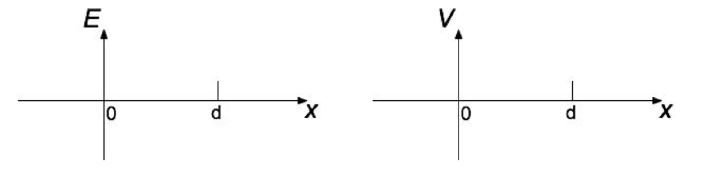Teaching Physics with the Physics Suite Edward F. Redish Problems Sorted by Type | Problems Sorted by Subject | Problems Sorted by Chapter in

Stuffing a capacitor

 One of the most important current problems in converting to "green energy" is storage. Lots of renewable energy resources – solar, wind – are intermittent and the times they are available don’t necessarily match when the energy is needed. One method of storage uses batteries but they are inefficient (a lot of the energy is lost) and expensive. An alternative being considered is the capacitor. This stores electrical energy in the electric fields between charges. Let's consider how this works and where the energy is. Two capacitors are shown in the figure at the right. In case A, the gap between the plates is air (which can be ignored). In case B, the gap is filled with a plastic insulator with dielectric constant κ (with κ > 1). This means that when both capacitor are charged to the same value Q, the electric field in between the plates in case B is reduced by a factor of κ compared to case A.A. In the two graphs below, sketch the electric field and the electrostatic potential that would be measured along the dotted line for capacitor A.B. The capacitor in A is connected across a battery for some time and then disconnected. The insulating slab is slid in between the plates as shown in B. How would the charge on the two plates of the capactior change and how would the potential between the two plates change? Explain.

C. Capacitors A and B are now connected across identical batteries. How would the voltage differences and the charges compare for the two cases? Explain your reasoning.

D. When both capacitors are charged to the same potential difference, ?V, which one would store more energy? Explain why.